|

# 梦想改造家|共助升级版美食之家,恒洁巧搭卫浴空间

连续参加四届《梦想改造家》以来都在小空间中施展「空间魔法」的史南桥老师终于有机会挑战120平方大户型了，但12根承重柱、20根横梁带来的空间凌乱，噪音大、空气差、无采光等造成的三代同堂居住难，恒洁梦改特约设计师史南桥如何让这些难题消弥于无形？

本期《舌尖上的家》中三代同堂的二胎之家已是当下中国的典型家庭结构，这种家庭结构对卫浴生活又有什么样的特殊期待？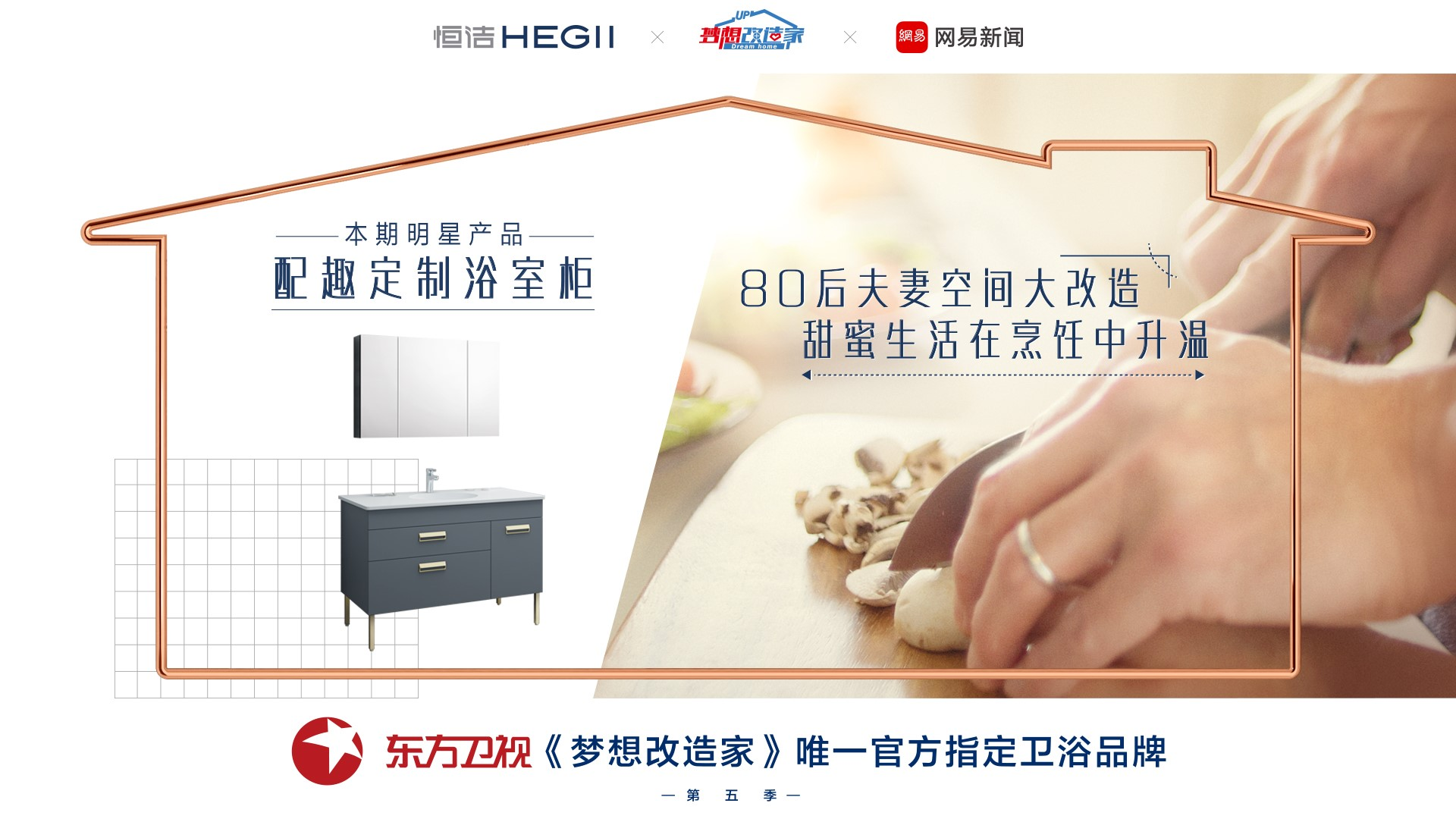在广州生活的小蔡和小青都是美食达人，有一对宝贝儿女。照说这样的家庭羡煞旁人。然而由于居住的是爷爷传下来的老式宿舍楼，虽有120平方米的空间，但12根承重柱、24根横梁让房屋结构很不合理，不但厨艺难尽情施展，就连去一趟卫生间，也必须穿过拥挤的厨房，这让全家分外尴尬。

此次改造，史南桥老师追求大道至简，把最好的人性化设计隐藏在细节里。史老师和施工团队克服了结构、环境、特殊空间需求等多重挑战，既满足了小蔡全家六口人实现全屋收纳、全屋隔音，通风、超美的中西开放式厨房……也为委托家庭和观众带来巨大惊喜。

梦改工程的结束，也意味着曾让小蔡家尴尬的卫浴生活彻底画上句号——自由、健康、便利、舒适的卫浴新生活从此开启。

卫浴间合二为一，功能强化升级

史南桥老师把卫浴间的升级作为改造重点。为让功能划分更合理，史南桥决定将原先一进门处的两个卫生间合二为一之后，移动到最东面，同时对卫生间格局进行了大刀阔斧的改变。改造后的卫浴空间，从原来只能一人使用的卫生间变成有两个台盆、两个坐便器以及同时具有冲淋和泡澡功能的浴室，既符合了业主只要一个卫生间的要求，同时也可以满足小蔡全家五个人同时使用而互不干扰。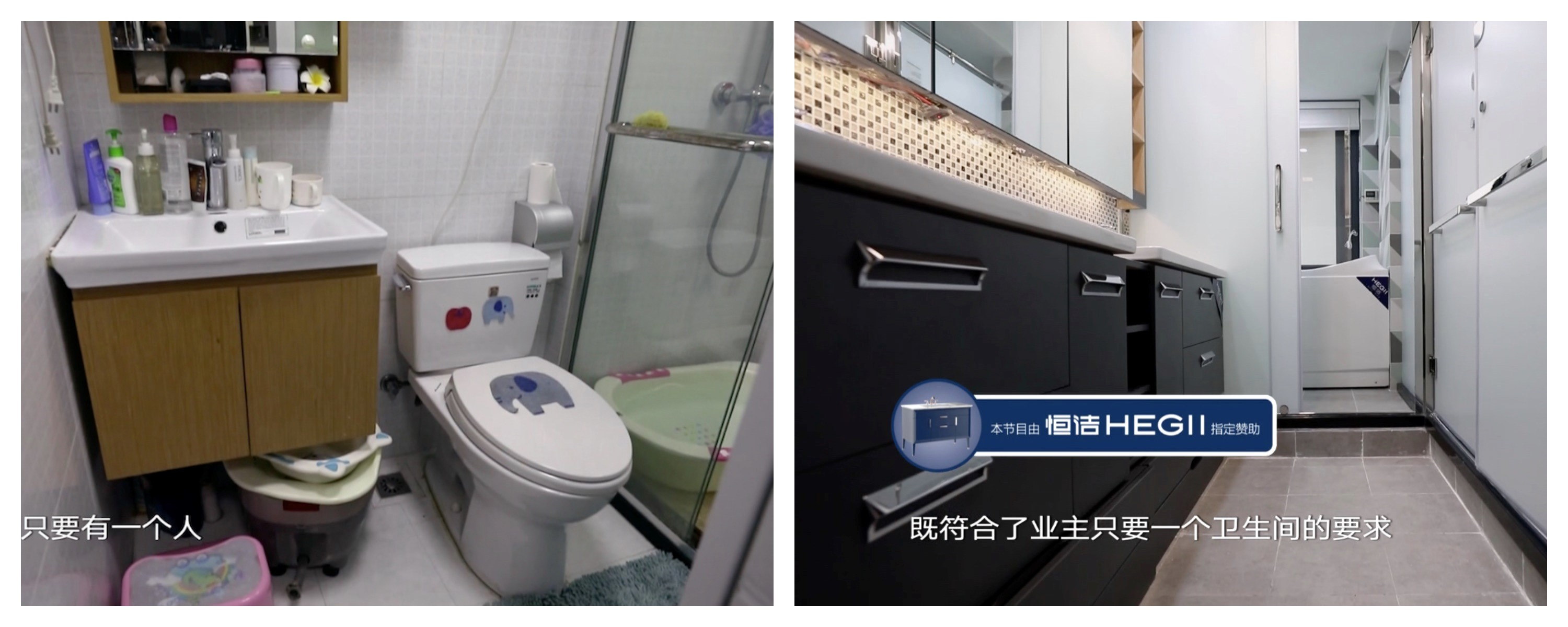▲ 卫浴间改造前后对比图，右为改造后。

恒洁配趣浴室柜，自由搭，随心配

原来的卫生间被委托人小青吐槽为「小巧玲珑」，有诸多不便。随着史南桥老师为委托人家庭定制的配趣浴室柜入驻，卫浴空间的使用更加舒适合理。配趣浴室柜强大的收纳功能，使得浴室空间更加整洁干净，也彰显出自由随性的生活方式。事实上，配趣浴室柜不仅是产品，它也在倡导「我的空间我做主」的卫浴空间个性化解决方案——多种颜色、材质、尺寸、柜体能够自由组合，与你的特别家居需求随心匹配。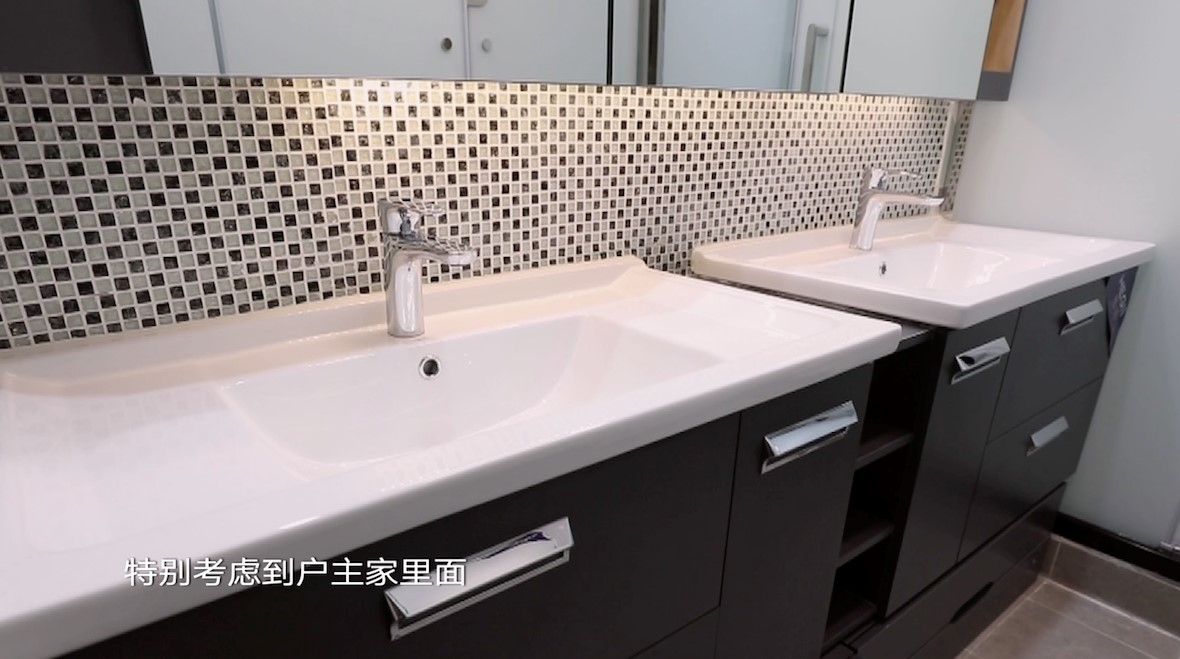为了委托人家庭两位小朋友的使用方便，本期梦改节目中的配趣浴室柜还特别设计了具有储存功能的踏板——从大人到小朋友，都能享受卫浴生活的乐趣。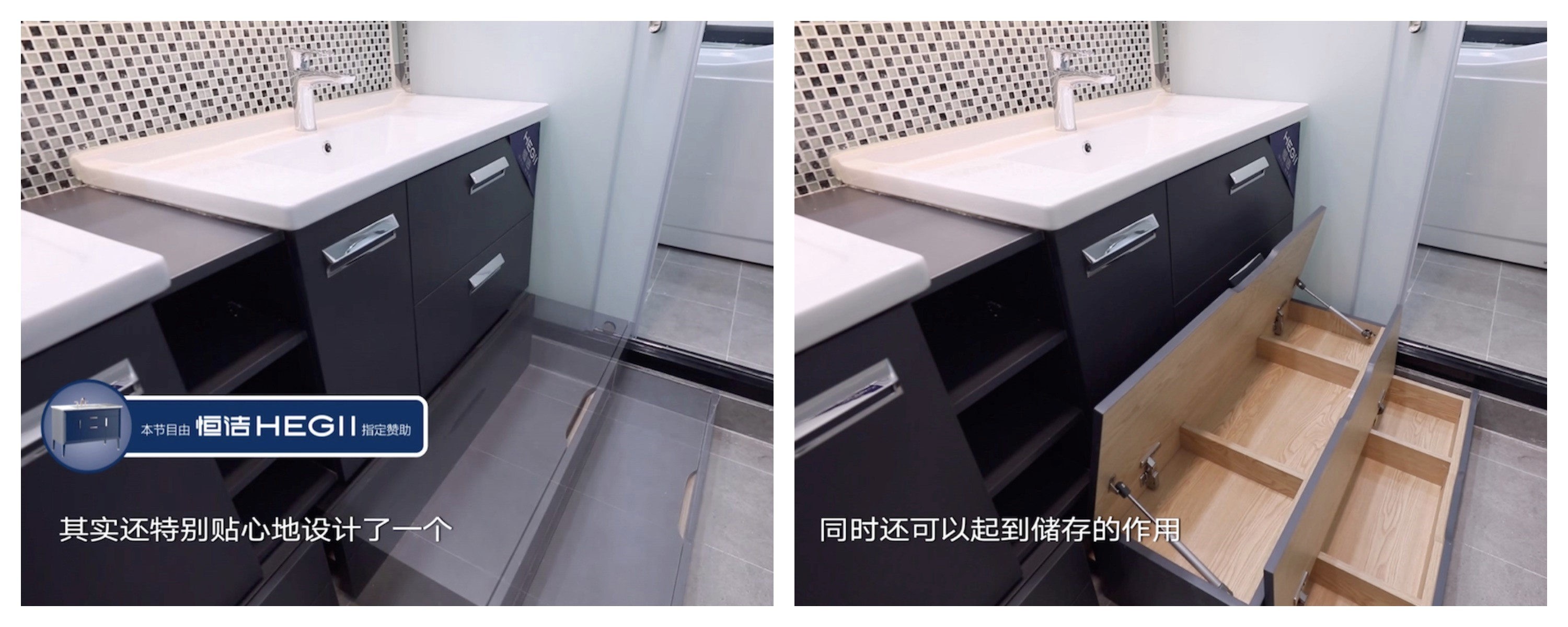恒洁乐氧花洒，环保中温柔以待

要全面照顾小蔡家三代六口人的淋浴需求，花洒产品必须同时满足环保、舒适、便捷等要求，因此，史南桥老师选择了恒洁乐氧大花洒产品。花洒独有的乐氧技术，能让约30%的空气和70%的水充分混合，增大水珠与身体的接触面，让使用者全身心侵润在春风细雨般的淋浴体验中。

另外，由于水和空气的混合，它能够比普通花洒有效节水30%。委托人小青曾吐槽为孩子洗澡时的诸多不便，现在通过乐氧花洒的一键切换专利技术，轻轻一按，即可随心切换不同出水效果，委托人全家今后的淋浴将变得更加简单舒适。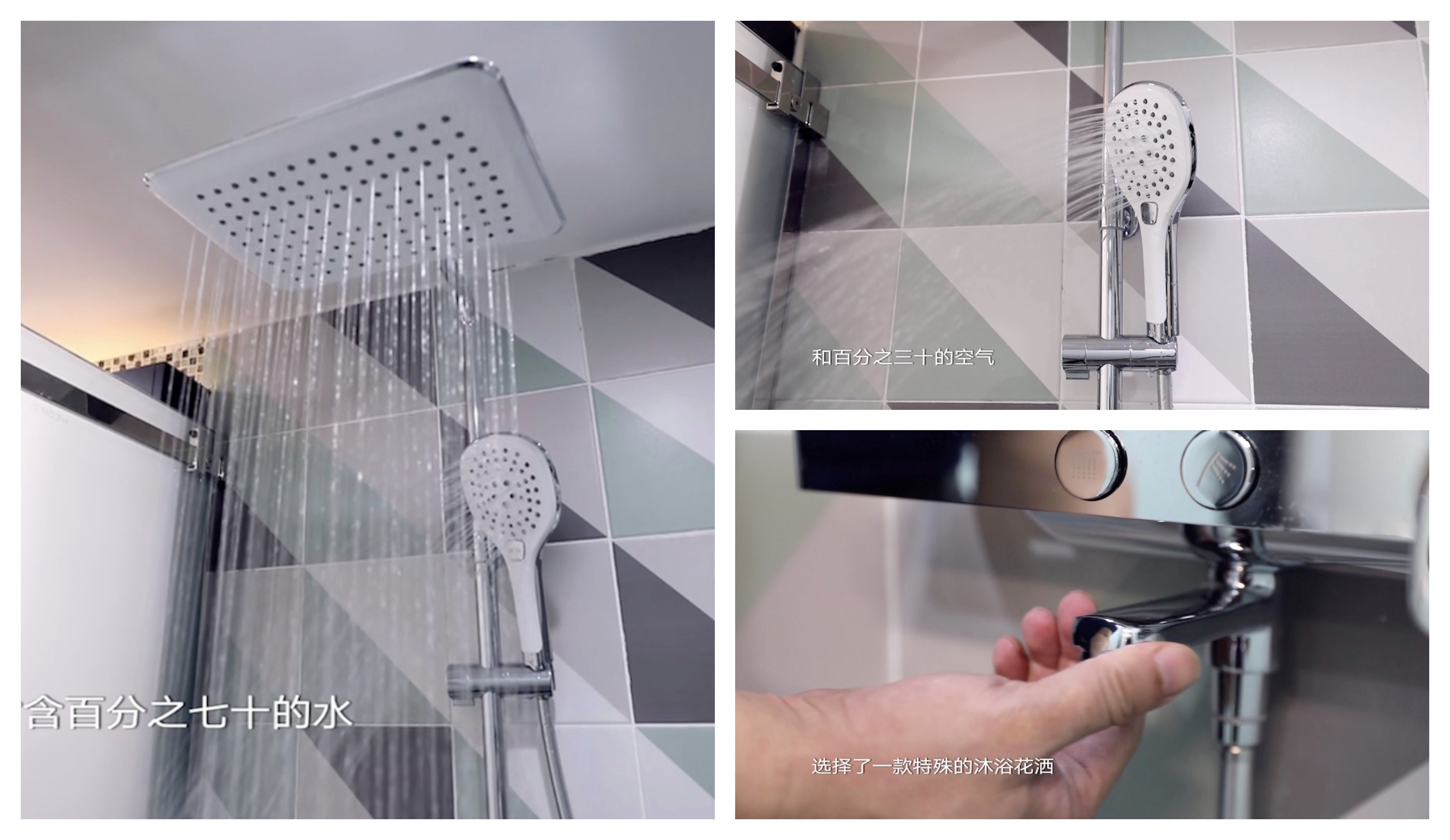恒洁节水坐便器，冲力强，更洁净

助力美好生活的还有恒洁节水坐便器——科学管道设计，不返流、不溅水、冲力强。它还秉承了恒洁陶瓷洁具固有的1260℃高温烧制品质，釉面光滑不黏污。坐便器特地采用了裙包管设计，更美观，也更易清洁。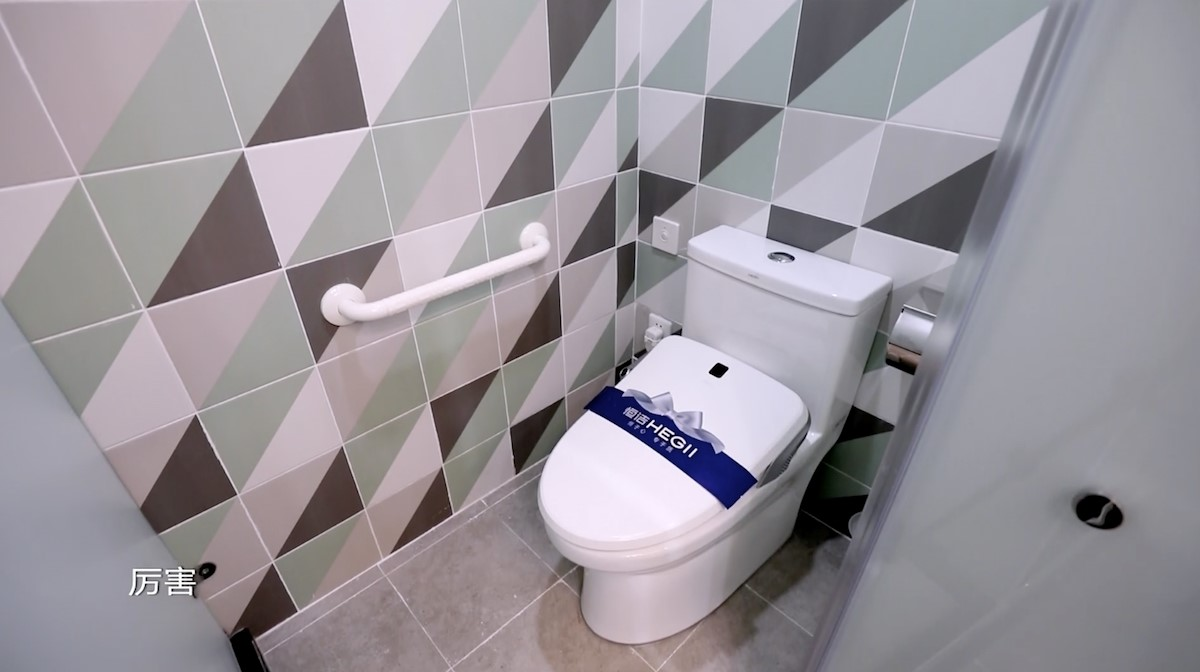恒洁浴缸，体感舒适，满足不同需求

在本期节目中使用的恒洁浴缸，灵动小巧，体感舒适，适合多种空间。这款浴缸还可坐可躺，满足不同需求，难怪小蔡妈妈一见倾心！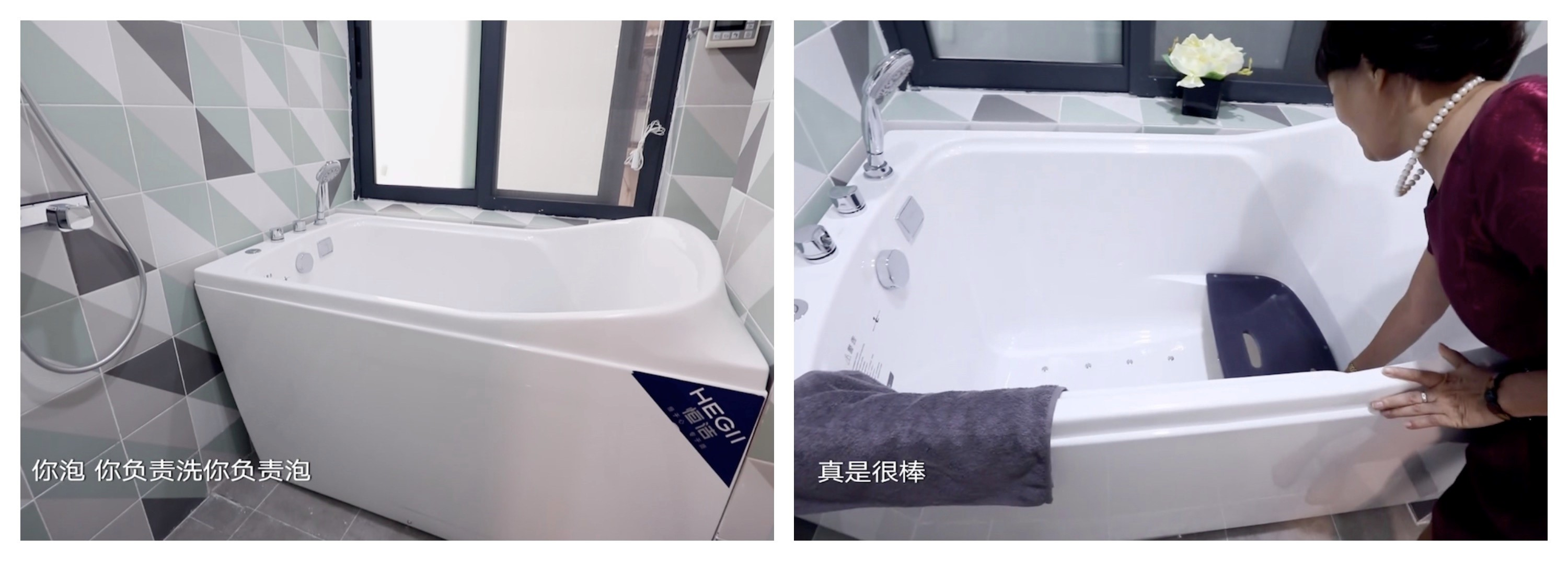下厨，不仅仅是为了吃饭，正如卫浴，也不仅仅是为了清洁。

在日复一日的现实生活中，总有一些更高的梦想值得我们一起去付出努力。恒洁相信，有趣的生活，不止于功能性的满足，而在于以自由和创新的精神，去挑战被禁锢的自我，与家人一起拥抱更美好的生活。

`声明：本文由入驻焦点开放平台的作者撰写，除焦点官方账号外，观点仅代表作者本人，不代表焦点立场错误信息举报电话： 400-099-0099，邮箱：jubao@vip.sohu.com，或点此进行意见反馈，或点此进行举报投诉。`A B C D E F G H J K L M N P Q R S T W X Y Z
A - B - C - D - E
• A
• 鞍山
• 安庆
• 安阳
• 安顺
• 安康
• 澳门
• B
• 北京
• 保定
• 包头
• 巴彦淖尔
• 本溪
• 蚌埠
• 亳州
• 滨州
• 北海
• 百色
• 巴中
• 毕节
• 保山
• 宝鸡
• 白银
• 巴州
• C
• 承德
• 沧州
• 长治
• 赤峰
• 朝阳
• 长春
• 常州
• 滁州
• 池州
• 长沙
• 常德
• 郴州
• 潮州
• 崇左
• 重庆
• 成都
• 楚雄
• 昌都
• 慈溪
• 常熟
• D
• 大同
• 大连
• 丹东
• 大庆
• 东营
• 德州
• 东莞
• 德阳
• 达州
• 大理
• 德宏
• 定西
• 儋州
• 东平
• E
• 鄂尔多斯
• 鄂州
• 恩施
F - G - H - I - J
• F
• 抚顺
• 阜新
• 阜阳
• 福州
• 抚州
• 佛山
• 防城港
• G
• 赣州
• 广州
• 桂林
• 贵港
• 广元
• 广安
• 贵阳
• 固原
• H
• 邯郸
• 衡水
• 呼和浩特
• 呼伦贝尔
• 葫芦岛
• 哈尔滨
• 黑河
• 淮安
• 杭州
• 湖州
• 合肥
• 淮南
• 淮北
• 黄山
• 菏泽
• 鹤壁
• 黄石
• 黄冈
• 衡阳
• 怀化
• 惠州
• 河源
• 贺州
• 河池
• 海口
• 红河
• 汉中
• 海东
• 怀来
• I
• J
• 晋中
• 锦州
• 吉林
• 鸡西
• 佳木斯
• 嘉兴
• 金华
• 景德镇
• 九江
• 吉安
• 济南
• 济宁
• 焦作
• 荆门
• 荆州
• 江门
• 揭阳
• 金昌
• 酒泉
• 嘉峪关
K - L - M - N - P
• K
• 开封
• 昆明
• 昆山
• L
• 廊坊
• 临汾
• 辽阳
• 连云港
• 丽水
• 六安
• 龙岩
• 莱芜
• 临沂
• 聊城
• 洛阳
• 漯河
• 娄底
• 柳州
• 来宾
• 泸州
• 乐山
• 六盘水
• 丽江
• 临沧
• 拉萨
• 林芝
• 兰州
• 陇南
• M
• 牡丹江
• 马鞍山
• 茂名
• 梅州
• 绵阳
• 眉山
• N
• 南京
• 南通
• 宁波
• 南平
• 宁德
• 南昌
• 南阳
• 南宁
• 内江
• 南充
• P
• 盘锦
• 莆田
• 平顶山
• 濮阳
• 攀枝花
• 普洱
• 平凉
Q - R - S - T - W
• Q
• 秦皇岛
• 齐齐哈尔
• 衢州
• 泉州
• 青岛
• 清远
• 钦州
• 黔南
• 曲靖
• 庆阳
• R
• 日照
• 日喀则
• S
• 石家庄
• 沈阳
• 双鸭山
• 绥化
• 上海
• 苏州
• 宿迁
• 绍兴
• 宿州
• 三明
• 上饶
• 三门峡
• 商丘
• 十堰
• 随州
• 邵阳
• 韶关
• 深圳
• 汕头
• 汕尾
• 三亚
• 三沙
• 遂宁
• 山南
• 商洛
• 石嘴山
• T
• 天津
• 唐山
• 太原
• 通辽
• 铁岭
• 泰州
• 台州
• 铜陵
• 泰安
• 铜仁
• 铜川
• 天水
• 天门
• W
• 乌海
• 乌兰察布
• 无锡
• 温州
• 芜湖
• 潍坊
• 威海
• 武汉
• 梧州
• 渭南
• 武威
• 吴忠
• 乌鲁木齐
X - Y - Z
• X
• 邢台
• 徐州
• 宣城
• 厦门
• 新乡
• 许昌
• 信阳
• 襄阳
• 孝感
• 咸宁
• 湘潭
• 湘西
• 西双版纳
• 西安
• 咸阳
• 西宁
• 仙桃
• 西昌
• Y
• 运城
• 营口
• 盐城
• 扬州
• 鹰潭
• 宜春
• 烟台
• 宜昌
• 岳阳
• 益阳
• 永州
• 阳江
• 云浮
• 玉林
• 宜宾
• 雅安
• 玉溪
• 延安
• 榆林
• 银川
• Z
• 张家口
• 镇江
• 舟山
• 漳州
• 淄博
• 枣庄
• 郑州
• 周口
• 驻马店
• 株洲
• 张家界
• 珠海
• 湛江
• 肇庆
• 中山
• 自贡
• 资阳
• 遵义
• 昭通
• 张掖
• 中卫

1室1厅1厨1卫1阳台

1
2
3
4
5

0
1
2

1

1

0
1
2
3报名成功，资料已提交审核A B C D E F G H J K L M N P Q R S T W X Y Z
A - B - C - D - E
• A
• 鞍山
• 安庆
• 安阳
• 安顺
• 安康
• 澳门
• B
• 北京
• 保定
• 包头
• 巴彦淖尔
• 本溪
• 蚌埠
• 亳州
• 滨州
• 北海
• 百色
• 巴中
• 毕节
• 保山
• 宝鸡
• 白银
• 巴州
• C
• 承德
• 沧州
• 长治
• 赤峰
• 朝阳
• 长春
• 常州
• 滁州
• 池州
• 长沙
• 常德
• 郴州
• 潮州
• 崇左
• 重庆
• 成都
• 楚雄
• 昌都
• 慈溪
• 常熟
• D
• 大同
• 大连
• 丹东
• 大庆
• 东营
• 德州
• 东莞
• 德阳
• 达州
• 大理
• 德宏
• 定西
• 儋州
• 东平
• E
• 鄂尔多斯
• 鄂州
• 恩施
F - G - H - I - J
• F
• 抚顺
• 阜新
• 阜阳
• 福州
• 抚州
• 佛山
• 防城港
• G
• 赣州
• 广州
• 桂林
• 贵港
• 广元
• 广安
• 贵阳
• 固原
• H
• 邯郸
• 衡水
• 呼和浩特
• 呼伦贝尔
• 葫芦岛
• 哈尔滨
• 黑河
• 淮安
• 杭州
• 湖州
• 合肥
• 淮南
• 淮北
• 黄山
• 菏泽
• 鹤壁
• 黄石
• 黄冈
• 衡阳
• 怀化
• 惠州
• 河源
• 贺州
• 河池
• 海口
• 红河
• 汉中
• 海东
• 怀来
• I
• J
• 晋中
• 锦州
• 吉林
• 鸡西
• 佳木斯
• 嘉兴
• 金华
• 景德镇
• 九江
• 吉安
• 济南
• 济宁
• 焦作
• 荆门
• 荆州
• 江门
• 揭阳
• 金昌
• 酒泉
• 嘉峪关
K - L - M - N - P
• K
• 开封
• 昆明
• 昆山
• L
• 廊坊
• 临汾
• 辽阳
• 连云港
• 丽水
• 六安
• 龙岩
• 莱芜
• 临沂
• 聊城
• 洛阳
• 漯河
• 娄底
• 柳州
• 来宾
• 泸州
• 乐山
• 六盘水
• 丽江
• 临沧
• 拉萨
• 林芝
• 兰州
• 陇南
• M
• 牡丹江
• 马鞍山
• 茂名
• 梅州
• 绵阳
• 眉山
• N
• 南京
• 南通
• 宁波
• 南平
• 宁德
• 南昌
• 南阳
• 南宁
• 内江
• 南充
• P
• 盘锦
• 莆田
• 平顶山
• 濮阳
• 攀枝花
• 普洱
• 平凉
Q - R - S - T - W
• Q
• 秦皇岛
• 齐齐哈尔
• 衢州
• 泉州
• 青岛
• 清远
• 钦州
• 黔南
• 曲靖
• 庆阳
• R
• 日照
• 日喀则
• S
• 石家庄
• 沈阳
• 双鸭山
• 绥化
• 上海
• 苏州
• 宿迁
• 绍兴
• 宿州
• 三明
• 上饶
• 三门峡
• 商丘
• 十堰
• 随州
• 邵阳
• 韶关
• 深圳
• 汕头
• 汕尾
• 三亚
• 三沙
• 遂宁
• 山南
• 商洛
• 石嘴山
• T
• 天津
• 唐山
• 太原
• 通辽
• 铁岭
• 泰州
• 台州
• 铜陵
• 泰安
• 铜仁
• 铜川
• 天水
• 天门
• W
• 乌海
• 乌兰察布
• 无锡
• 温州
• 芜湖
• 潍坊
• 威海
• 武汉
• 梧州
• 渭南
• 武威
• 吴忠
• 乌鲁木齐
X - Y - Z
• X
• 邢台
• 徐州
• 宣城
• 厦门
• 新乡
• 许昌
• 信阳
• 襄阳
• 孝感
• 咸宁
• 湘潭
• 湘西
• 西双版纳
• 西安
• 咸阳
• 西宁
• 仙桃
• 西昌
• Y
• 运城
• 营口
• 盐城
• 扬州
• 鹰潭
• 宜春
• 烟台
• 宜昌
• 岳阳
• 益阳
• 永州
• 阳江
• 云浮
• 玉林
• 宜宾
• 雅安
• 玉溪
• 延安
• 榆林
• 银川
• Z
• 张家口
• 镇江
• 舟山
• 漳州
• 淄博
• 枣庄
• 郑州
• 周口
• 驻马店
• 株洲
• 张家界
• 珠海
• 湛江
• 肇庆
• 中山
• 自贡
• 资阳
• 遵义
• 昭通
• 张掖
• 中卫• 手机• 分享
• 设计
免费设计
• 计算器
装修计算器
• 入驻
合作入驻
• 联系
联系我们
• 置顶
返回顶部